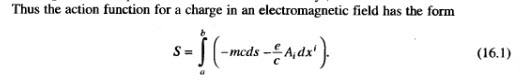# Variational principle & lorentz force law

## Homework Statement

Show that the Lorentz force law follows from the following variational principle:
$$S=\frac{m}{2}\int\eta_{\mu\nu}u^\mu u^\nu ds-q\int A_\mu u^\mu ds$$

## Homework Equations

Definition of Field Strength Tensor
Integration by Parts
Chain Rule & Product Rule for Derivatives

## The Attempt at a Solution

In order to find the force equation, we need to vary the action and find when $\delta S=0$.

So, we begin by varying the action integral,
$$\delta S=\frac{m}{2}\int\eta_{\mu\nu}\delta(u^\mu u^\nu)ds-q\int\delta(A_\mu u^\mu)ds$$

Then apply product rule to expand all the terms,
$$\delta S=m\int\eta_{\mu\nu}u^\nu\frac{d(\delta x^\mu)}{ds}ds-q\int\left(\partial_\nu A_\mu u^\mu\delta x^\nu+A_\mu\frac{d(\delta x^\mu)}{ds}\right)ds$$

Simplified and renamed indices in the second term,
$$\delta S=m\int u_\mu\frac{d(\delta x^\mu)}{ds}ds-q\int\left(\partial_\mu A_\nu u^\nu\delta x^\mu+A_\mu\frac{d(\delta x^\mu)}{ds}\right)ds$$

Reverse integration by parts to get the variation out of the derivatives,
$$\delta S=m\int\left(\frac{d}{ds}\left(u_\mu\delta x^\mu\right)-\frac{du_\mu}{ds}\delta x^\mu\right)ds-q\int\left(\partial_\mu A_\nu u^\nu\delta x^\mu+\frac{d}{ds}\left( A_\mu\delta x^\mu\right)-\frac{dA_\mu}{ds}\delta x^\mu\right)ds$$

Grouping like terms we have,
$$\delta S=\int\left(\frac{d}{ds}\left(mu_\mu\delta x^\mu-qA_\mu\delta x^\mu\right)\right)ds+\int \left( q\left(\frac{dA_\mu}{ds}\delta x^\mu-\partial_\mu A_\nu u^\nu\delta x^\mu\right)-m\frac{du_\mu}{ds}\delta x^\mu\right)ds$$

After integration, the first term should be zero since the variations must vanish at the endpoints. Also, using the chain rule on the dA/ds derivative gives me
$$\delta S=\int \left( q\left(\partial_\nu A_\mu u^\nu-\partial_\mu A_\nu u^\nu\right)-m\frac{du_\mu}{ds}\right)\delta x^\mu ds$$

Rearranged terms to simplify further,
$$\delta S=\int \left( -q\left(\partial_\mu A_\nu-\partial_\nu A_\mu\right)u^\nu -m\frac{du_\mu}{ds}\right)\delta x^\mu ds$$

Then I use the definition of the field strength tensor to get,
$$\delta S=\int \left( -qF_{\mu\nu}u^\nu -m\frac{du_\mu}{ds}\right)\delta x^\mu ds$$

Then $\delta S=0$ for any arbitrary variation $\delta x^\mu$ only if the integrand itself is zero, so
$$qF_{\mu\nu}u^\nu+m\frac{du_\mu}{ds}=0$$

Rearranging the expression gives
$$\frac{du_\mu}{ds}=-\frac{q}{m}F_{\mu\nu}u^\nu$$

This is the correct form for the Lorentz force law, however I have an additional minus sign. It's probably a trivial problem, but I've double checked all the work several times and I can't figure out where it's coming from.

Last edited:

## Answers and Replies

Perhaps the problem is this:

On the right hand side you have $$u^\nu$$
On the left hand side $$u_\mu$$

The relation between the two is given by $$\eta_{\mu\nu}$$ and that depends on your signature convention. This signature is expressed in the sign of the first term of your action principle.

I checked my notes and it's supposed to come out to
$$\frac{du_\mu}{ds}=\frac{q}{m}F_{\mu\nu}u^\nu$$

We've been using the convention that the spatial terms have the minus signs in the Minkowski metric (+---). In an earlier step I used $u_\mu=\eta_{\mu\nu}u^\nu$ to get the lower mu index in the first term with the mass. I can't find anything that says how the signature affects the process of raising/lowering indices though.

Question is why do you have minus sign in front of the second (or plus in front of the first) term in:

$$S=\frac{m}{2}\int\eta_{\mu\nu}u^\mu u^\nu ds-q\int A_\mu u^\mu ds$$

Landau and Lifgarbagez has this actionThe first term is $$\sqrt{\eta_{\mu\nu}u^{\mu}u^{\nu}}\,ds$$ but it should not matter.

Last edited: